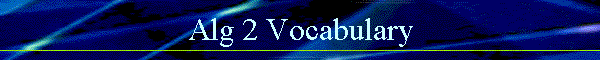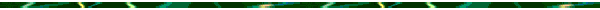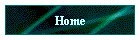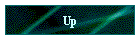Algebra 2 Vocabulary Words By Chapter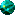Chapter 1  Basic Concepts of AlgebraChapter 2  Inequalities and ProofChapter 3  Linear Equations and FunctionsChapter 4  Products and Factors of PolynomialsChapter 5  Rational ExpressionsChapter 6  Irrational and Complex NumbersChapter 7  Quadratic Equations and FunctionsChapter 8  Variation and Polynomial EquationsChapter 9  Analytic GeometryChapter 10  Exponential and Logarithmic FunctionsChapter 11  Sequences and SeriesChapter 12 Triangle TrigonometryChapter 13  Trigonometric Graphs; IdentitiesChapter 14  Trigonometric ApplicationsChapter 15  Statistics and ProbabilityChapter 16  Matrices and Determinants     (Ch 16 Word List)